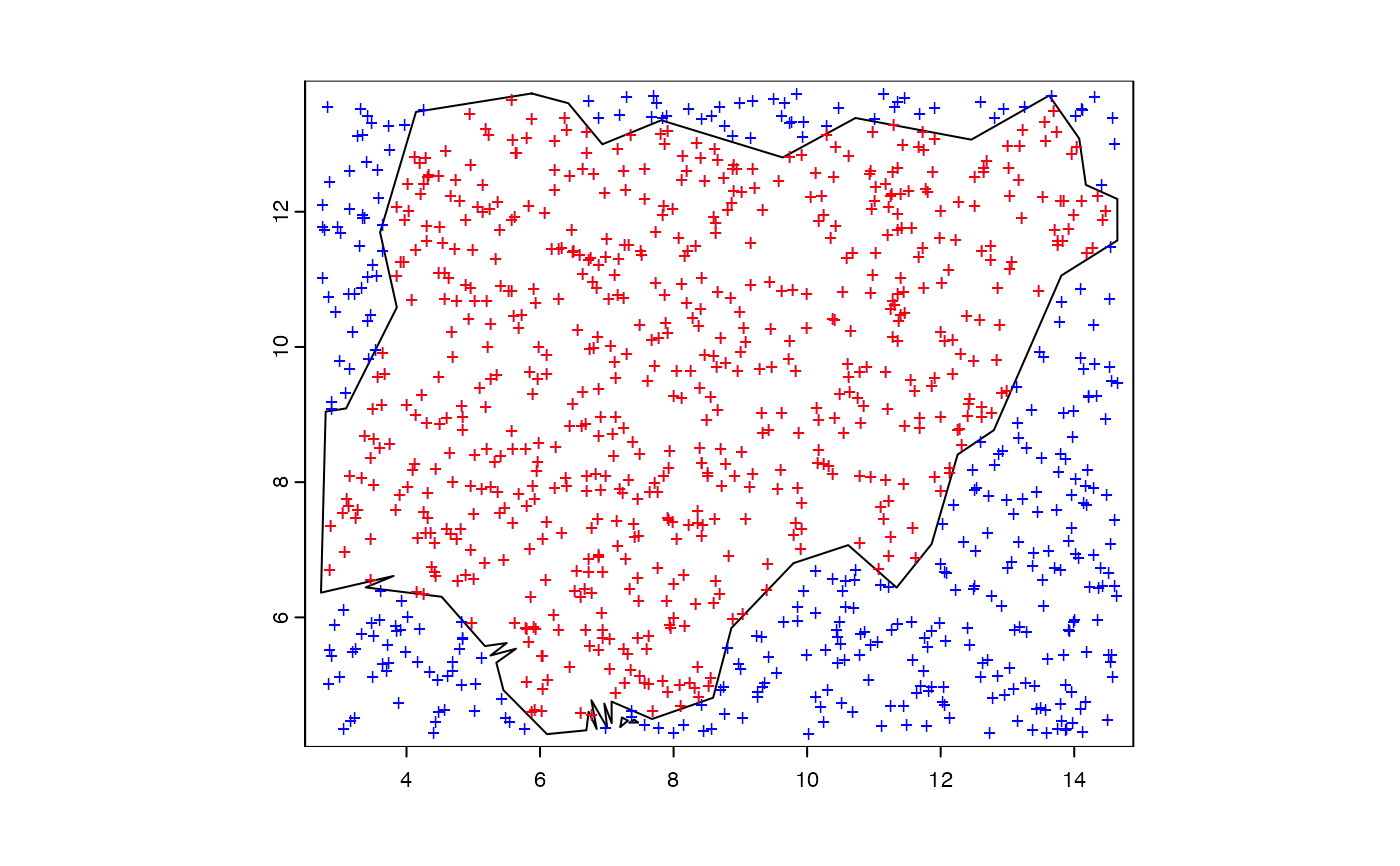The selectbylocation function selects features based on their location relative to features in another layer.

selectbylocation(x, y)

## Arguments

x

source layer of the class SpatVect

y

Target layer or mask extent to subset from.

## Value

A spatial polygons or spatial points object pruned to the extent of the target layer.

## Examples

library(terra)
d <- vect(system.file("ex/nigeria.json", package="phyloregion"))
e <- ext(d)

set.seed(1)
m <- data.frame(lon = runif(1000, e, e),
lat = runif(1000, e, e),
sites = seq(1000))
m <- vect(m)
z <- selectbylocation(m, d)
plot(d)
points(m, col = "blue", pch = "+")
points(z, col = "red", pch = "+")